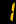# IBDP

### Mathematical studies SL

This course caters for students with varied backgrounds and abilities. More specifically, it is designed to build confidence and encourage an appreciation of mathematics in students who do not anticipate a need for mathematics in their future studies. Topics will be taught:

1. The graphic display calculator
2. Number and algebra
3. Sets, logic, and probability
4. Functions
5. Geometry and trigonometry
6. Statistics
7. Introductory differential calculus
8. Financial mathematics

### Mathematics SL

This course caters for students who already possess knowledge of basic mathematical concepts, and who are equipped with the skills needed to apply simple mathematical techniques correctly. The majority of these students will expect to need a sound mathematical background as they prepare for future studies in subjects such as chemistry, economics, psychology and business administration. Topics will be taught:

1. Algebra
2. Functions and equations
3. Circular functions and trigonometry
4. Vectors
5. Statistics and probability
6. calculus

### Learning Objectives

Students will know and use mathematical concepts and principles. In particular, students must be able to:

• read, interpret, and solve a given problem using appropriate mathematical terms
• organize and present information and data in tabular, graphical and/or diagrammatic forms
• know and use appropriate notation and terminology
• formulate a mathematical argument and communicate it clearly
• select and use appropriate mathematical strategies and techniques
• demonstrate an understanding of both the significance and the reasonableness of results
• recognize patterns and structures in a variety of situations, and make generalizations
• recognize and demonstrate an understanding of the practical applications of mathematics
• use appropriate technological devices as mathematical tools
• demonstrate an understanding of and the appropriate use of mathematical modeling.